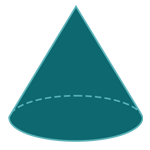Report a Problem
Suggestions

#Calculate The Surface Area of A Cone

Last updated: Saturday, April 29, 2023
Select a type of cone below
Right Cone
Truncated Cone

The surface area of a cone is the sum of the curved surface area and the base area. The curved surface area of a cone is the lateral area, which is the area of the cone excluding the base. The base area of a cone is simply the area of the circle that forms its base.

Real-life examples of cones include traffic cones, ice cream cones, and the shape of a volcano. Calculating the surface area of a cone is useful in various fields, such as engineering, architecture, and construction. For example, when designing a traffic cone, the surface area is important to ensure the cone is visible and stable. In architecture, the surface area of a cone-shaped roof is considered to determine the amount of materials needed for construction.

The formula for determining the surface area of a cone is defined as:
$$SA$$ $$=$$ $$\pi$$ $$\cdot$$ $$r$$ $$\cdot$$ $$l$$ $$+$$ $$\pi$$ $$\cdot$$ $$r^2$$
$$SA$$: the surface area of the cone
$$r$$: the radius of the base
$$l$$: the length of the slant height
The SI unit of surface area is: $$square \text{ } meter\text{ }(m^2)$$
Select a problem below
Find $$SA$$
Find $$SA$$

## Find $$SA$$

Use this calculator to determine the surface area of a cone when the length of the slant height and the radius of the base are given.
Hold & Drag
CLOSE
$$r$$
$$meter$$
$$l$$
$$meter$$